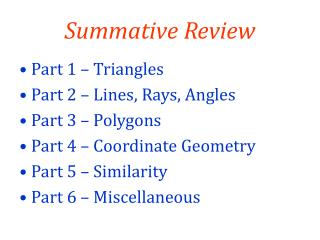DownloadDownload PresentationSummative Review

# Summative Review

Télécharger la présentation## Summative Review

- - - - - - - - - - - - - - - - - - - - - - - - - - - E N D - - - - - - - - - - - - - - - - - - - - - - - - - - -
##### Presentation Transcript

1. Summative Review • Part 1 – Triangles • Part 2 – Lines, Rays, Angles • Part 3 – Polygons • Part 4 – Coordinate Geometry • Part 5 – Similarity • Part 6 – Miscellaneous

2. Please Take Out Worksheet From Yesterday and Continue Working! Grab your calculators if needed! (We will go over Part 1 Today)

3. Part 1 – Triangles

4. 14. The angle of inclination from an ants eye to the top of 75ft building is 42°, how far is the ant from the building? 15. An equilateral triangle has a side length of 6cm. Find the area of the triangle. Draw a picture and show your computations.

5. Part 2 – Lines, Rays, Angles

6. Part 3 – Polygons • Find the sum of the measures of the interior angles • of a regular hexagon. **2. Find the measure of an interior angle of a regular pentagon. Show or explain your work. 3. What is the sum of the measures of the exterior angles of any polygon?

7. Which convex polygon’s interior angles has the same • sum as the exterior angles? How do you know? **5. Find the measure of an exterior angle of a regular octagon. Show or explain your work.

8. Matt says that every quadrilateral with congruent • diagonals is a square. Name a figure that can • be used to disprove Matt’s statement. • Which quadrilaterals could be classified as • parallelograms? (trapezoid, rhombus, square, • kite, rectangle)

9. Part 4 – Coordinate Geometry 1) a) If triangle TRY is REFLECTED, what are the coordinates of Y? b) If triangle TRY is TRANSLATED, what are the coordinates of Y?

10. 2) A circle is drawn on a grid. The endpoints of a diameter of the circle are (-2,17) and (8, 11). What are the coordinates of the center of the circle?

11. **3) Given the diagram: a) Write an equation of a line that is PARALLEL to the line below and through the point k. b) Write an equation of a line that is PERPENDICULAR to the line below and through k.

12. Part 5 – Similarity • In the figure on the right, • ABCD is similar to DGFE. • Find the length of CD.

13. 2. Two circles have radii in the ratio 3:2. The larger circle has radii 12, what is the circumference of the smaller circle?

14. **3. In the figures below, ABCDE is similar to HIJKL, what is the length of HI? What is the perimeter of HIJKL?

15. Part 6 – Miscellaneous • In the figure shown, <MQN is congruent to <POQ • and segment NQ is congruent to segment OQ. Explain why ΔMNQ is congruent to ΔPOQ. Use geometric theorems or postulates in your explanation.

16. A signed Jermaine Dye 2005 World Series baseball • is in a cubic display box. The ball has radius 3 inches • and is snug in the box. What is the volume of space in • the box that is not being occupied by Dye’s ball?